# Steppi: An information-based Change-Point Analysis tool for biophysical applications implemented in MATLAB.

Colin H. LaMont and Paul A. Wiggins

Abstract: Change-point analysis is a flexible and computationally tractable tool for the analysis of times series data from systems that transition between discrete states and whose observables are corrupted by noise. The change-point algorithm is used to identify the change-points, the times at which the system transitions between states and fit the model parameters in each state. We present a unified approach to the analysis of processes whose noise can be modeled by Gaussian, Wiener or Ornstein-Uhlenbeck Processes. Using explicit, closed-form algebraic expressions for maximum-likelihood estimators of model parameters and estimated information loss of the generalized noise model that can be computed extremely efficiently. Next, we demonstrate that a Change-Point Analysis can be implemented using a single statistical test (Frequentist Information Criterion) that depends on the number of parameters fit per state and the number of observations. This approach reconciles two previously disparate approaches to Change-Point Analysis (test-statistic and model-selection criterion) for testing transitions between states. The use of the information criterion significantly simplifies the statistical analysis and facilitate the the calculation of explicit expressions for the resolution of the technique for determining small changes in the model parameters. We expect this technique to be of general interest to experimental investigators interested in biological systems. Applications of this analysis include molecular-motor stepping, fluorophore bleaching, electrophysiology, particle and cell tracking, detection of copy number variation by sequencing, tethered- particle-motion etc.

References: Biophysical Journal and Neural Computation.

How change-point analysis works: The change-point algorithm is used to identify the change-points, the times at which the system transitions between states and fit the model parameters in each state. The parameters describing each state are assumed to be stationary (i.e. not changing in time). The time evolution of the signal is represented by transitions between the states. In our biophysical implementation, we parameterize the state signal with four types of parameters, illustrated in the figure below:

 Parameter Name Symbol Description of an application level mean $\mu$ Position, intensity, copy number, ... level slope $\alpha$ Drift velocity stiffness/variance $k$ Diffusion coefficient, coupling $\epsilon$ Spring stiffness in Tethered Particle Motion (TPM)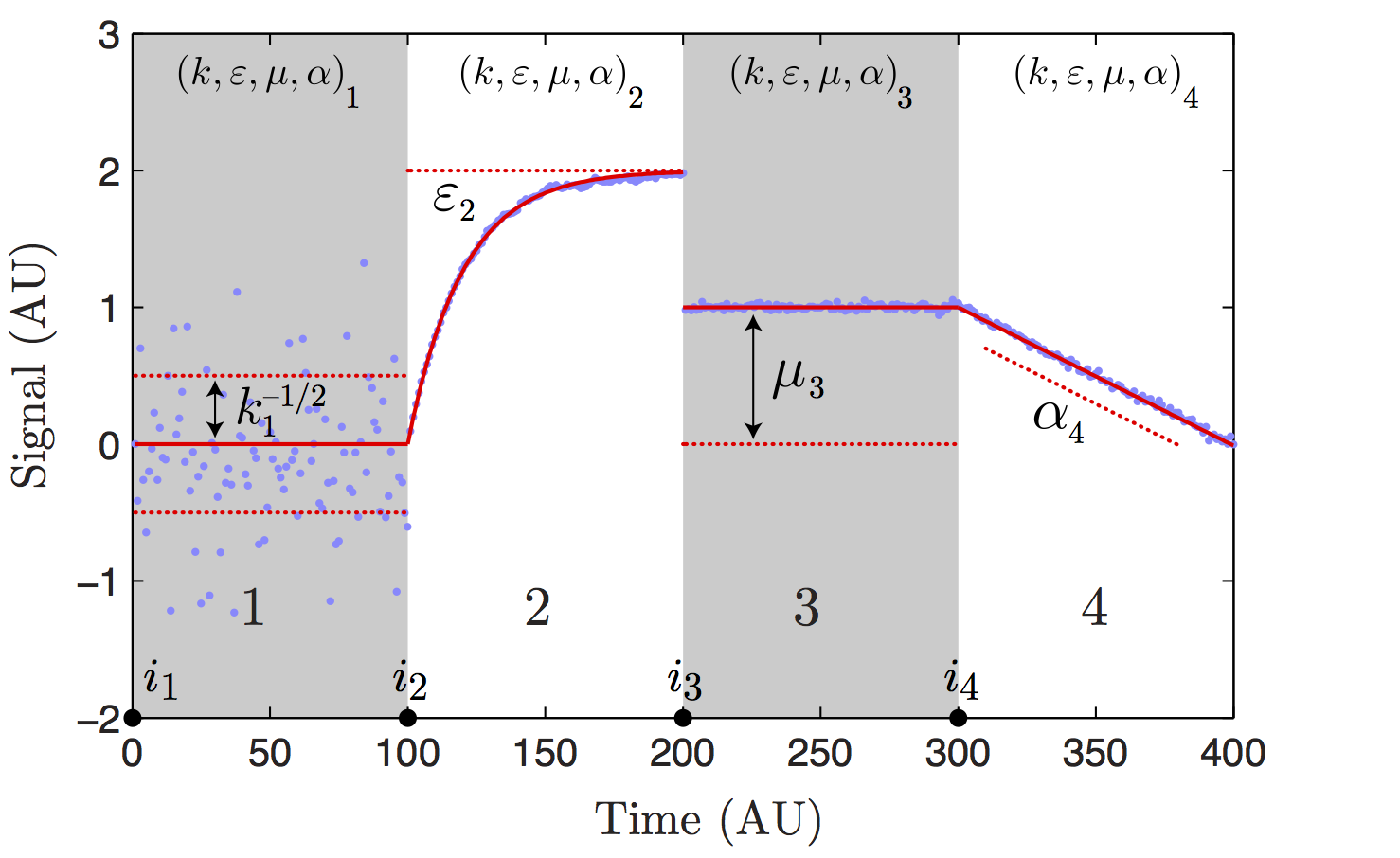State model schematic. The state model signal is characterized by four model parameters that are written as the vector θ ≡ (k, ε, μ, α). Above we schematically illustrate the role of each parameter in shaping the signal. The parameter k parameterizes the standard deviation of the noise (σ = k^−1/2). State two illustrates the effect of the finite lifetime of fluctuations in models with autoregression (0 < ε < 1). State three illustrates the role of the level mean μ. State four illustrates of the role of the level slope (α).

There are three choices for each of these parameters: (i) They may be set by hand, (ii) They may be chosen to have an unknown but global value (i.e. shared between all states) or (iii) they may have an unknown but local value (determined for each state independently.)

# Examples of the Steppi Package

(1) Wiener Process: Drift Diffusion As an example of a Wiener Process, we present the example of diffusion with a bias in one dimension. (The code works in higher dimension as well.) We simulated data so that the true model is known. The system transitions back and forth between states with diffusion coefficients D = { 0.25, 2.5 x 10^-3}/2. The state with the lower diffusion constant has a small drift velocity with a random orientation.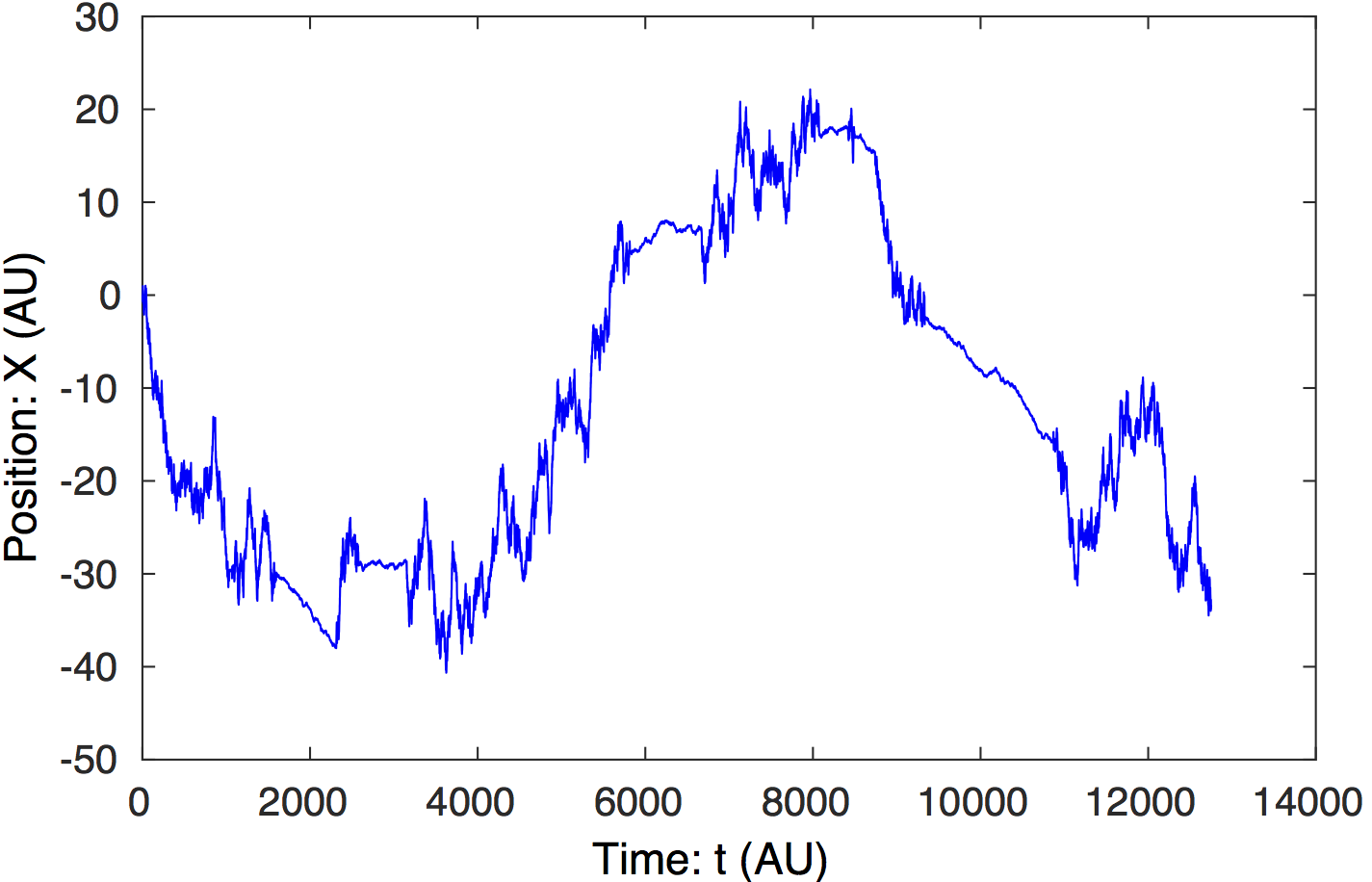Raw data. The raw particle trajectory is shown above. The transitions between states with large and small diffusion coefficients are clearly visible. (E.g. a transition occurs at t = 1,600.)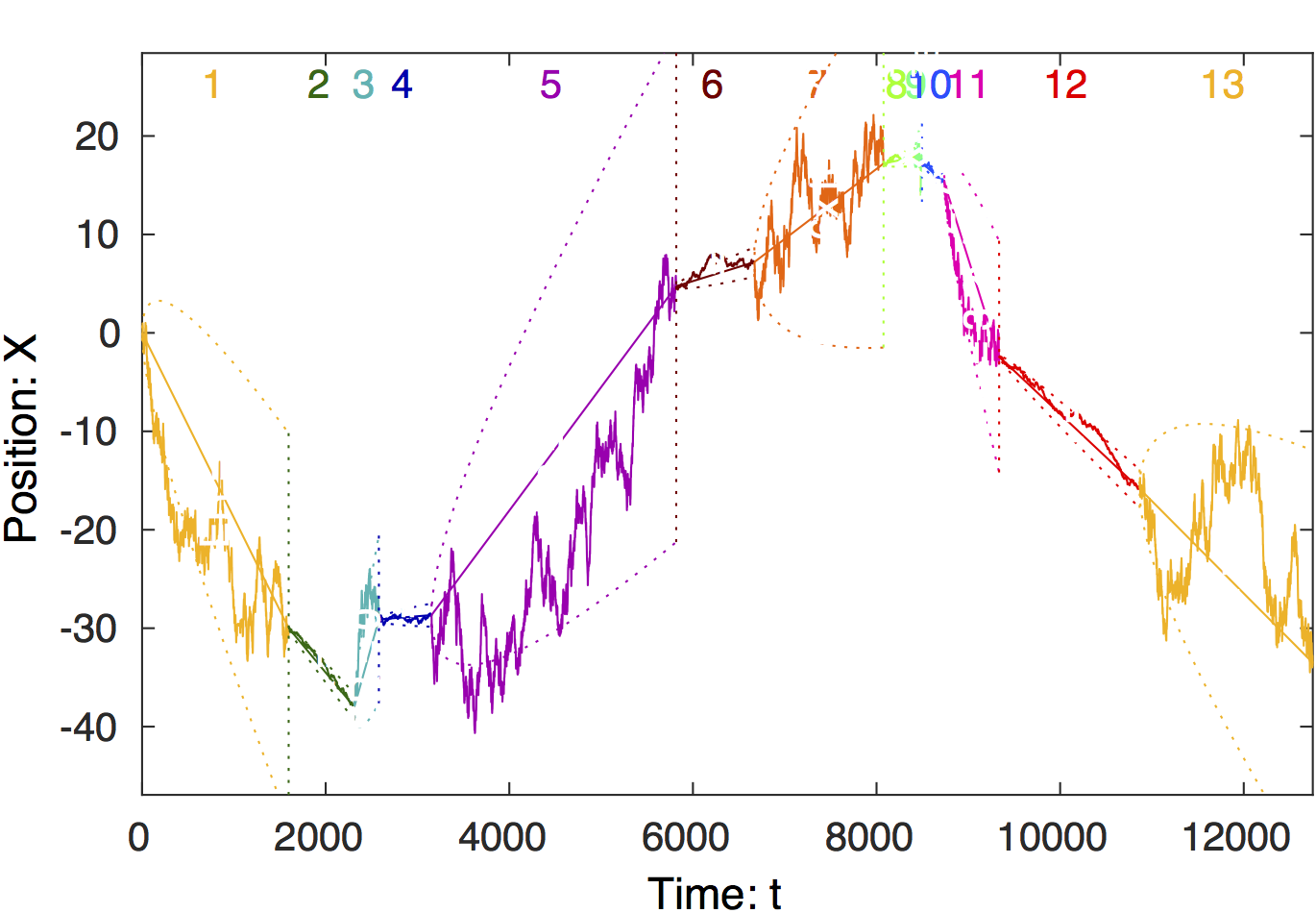Change-point analysis of signal. Steppi determines the positions of the change points and fits the model parameters (a diffusion constant (i.e. stiffness $k = \sigma^\left\{-2\right\}$) and drift velocity (i.e. level slope $\alpha$) in each state. The trajectory is colored by state with the state number shown at the top of the figure. The true number of states is recovered (n = 13).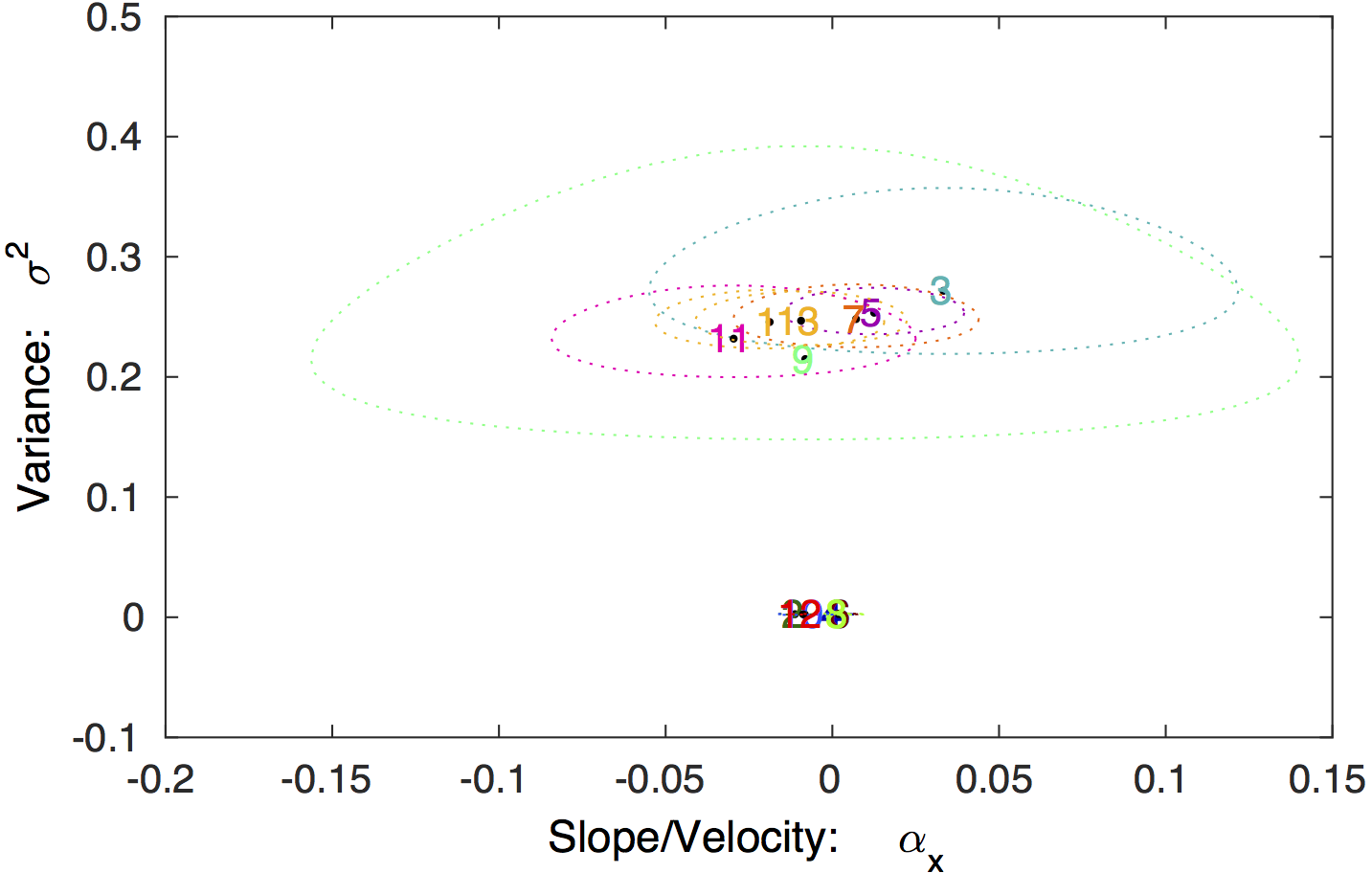Model parameter values. The 95% confidence regions for the model parameters for each state is shown, in addition to the MLE values. The true diffusion constants are $\sigma^2 =$2D = { 0.25, 2.5 x 10^-3}, in excellent agreement with the analysis.

### (2) Gaussian Process: Motor stepping

As an example of a Gaussian Process, we present the example of motor stepping. Again, we simulate the data so that the true distribution is known. In the change-point analysis, we treat the motor position as the level mean with an unknown position (unique for  each step) and a global unknown stiffness.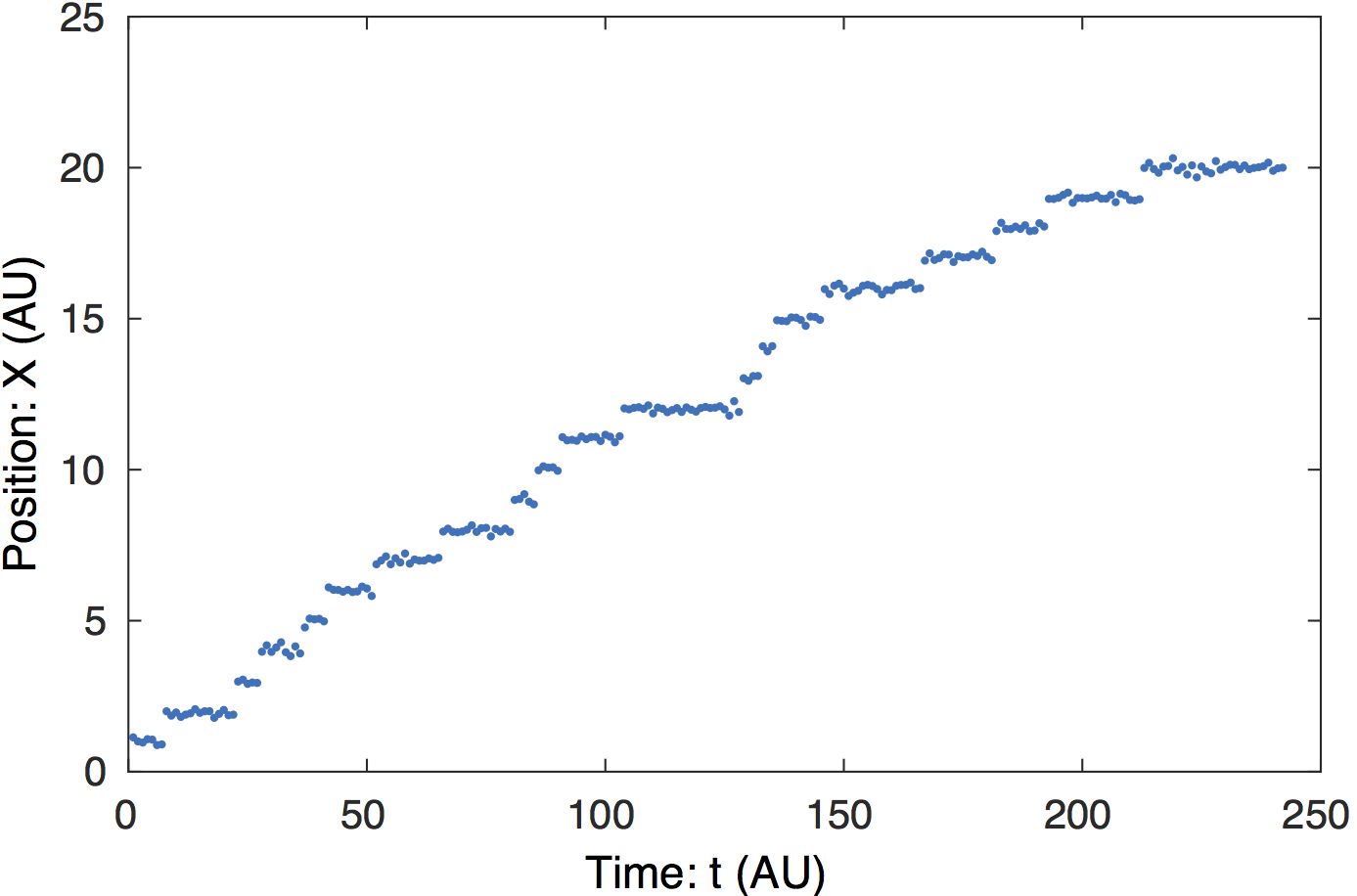Raw data.  In the simulated data, the step length is 1. The step-like transitions are clear by eye.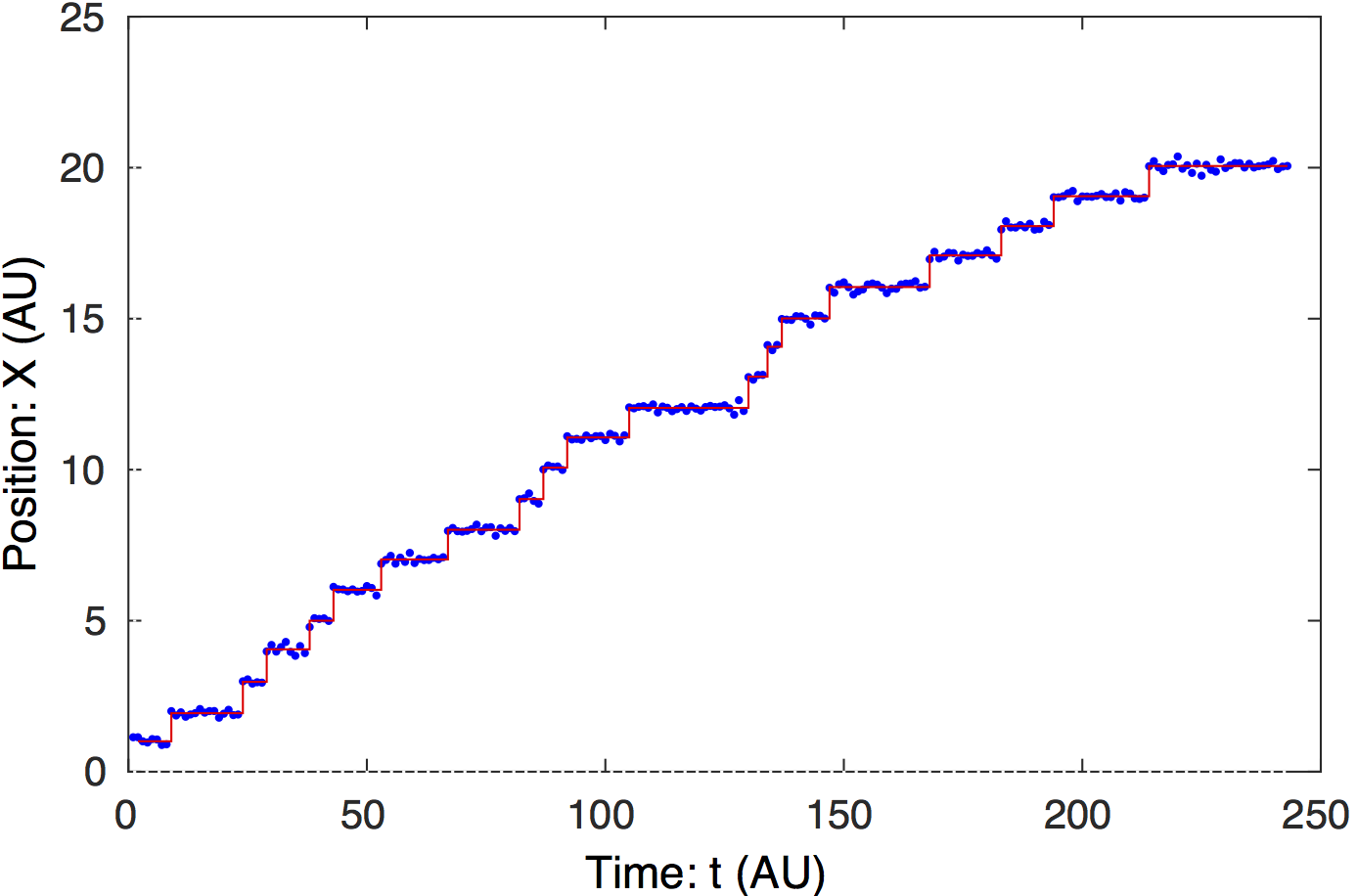Change-point analysis of signal. Steppi determines the positions of the change points and fits the model parameters (level mean $\mu$) and global stiffness ($k$).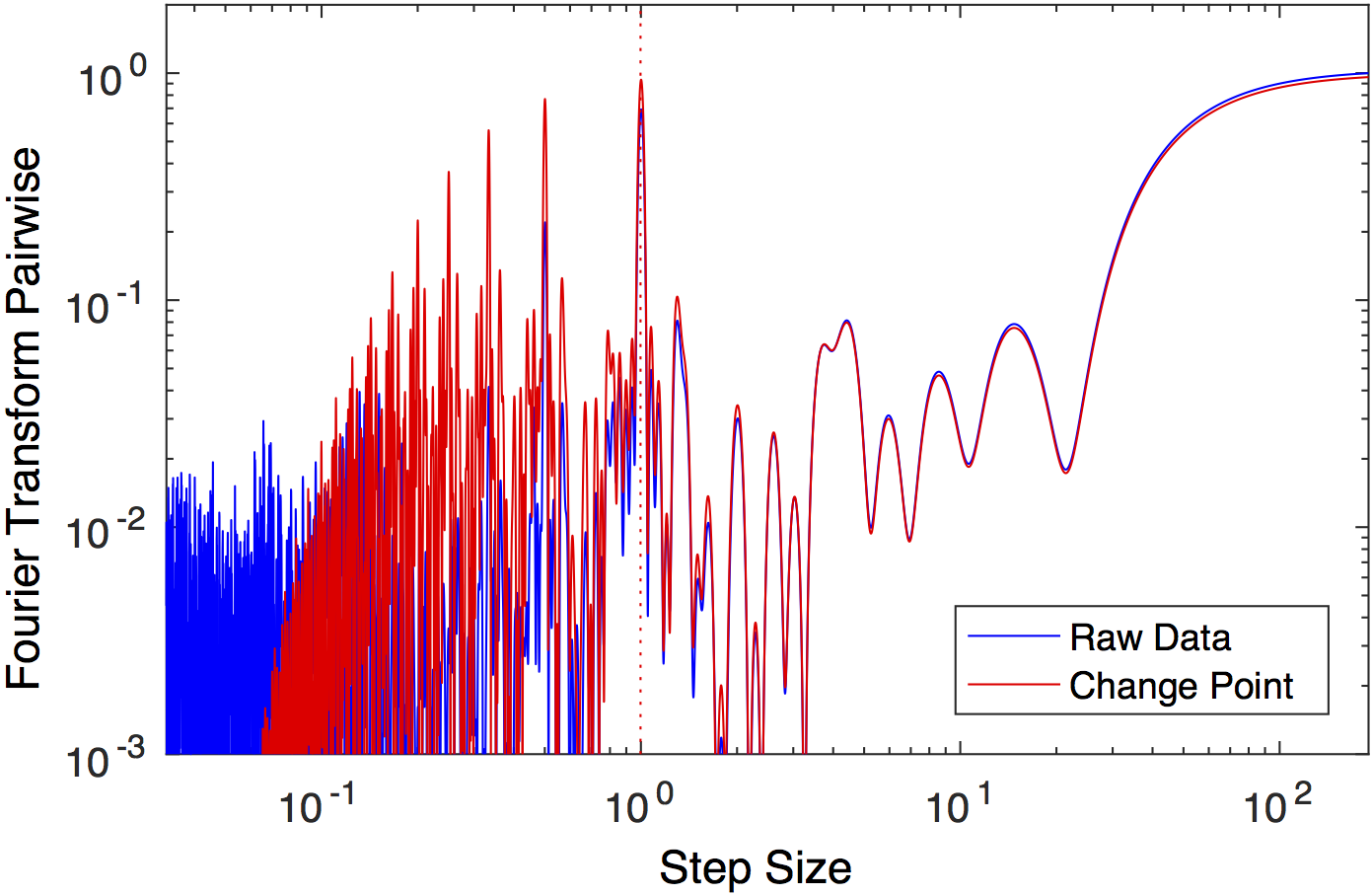Fourier transform of the pairwise distribution function. A dotted line shows the peak with the greatest power, corresponding to the unitary step length, in excellent agreement with the simulated stepsize 1.

### (3) Ornstein-Uhlenbeck Process: Tether Particle Motion

As an example of an Ornstein-Uhlenbeck Process, we present the example of the analysis of Tethered-Particle-Motion. In short, a bead diffuses on a a DNA tether. The DNA tether is approximated by a linear spring. The motion of the bead is therefore subject to three driving forces: (i) thermal fluctuations, (ii) damping forces from viscosity and (iii) forces from the deformation of the DNA tether.

On short time scales, the system behaves like a Wiener Process: dominated by thermal fluctuations and viscus damping forces. On long time scales, the system behaves like a Gaussian Process: dominated by thermal fluctuations and forces from the DNA tether.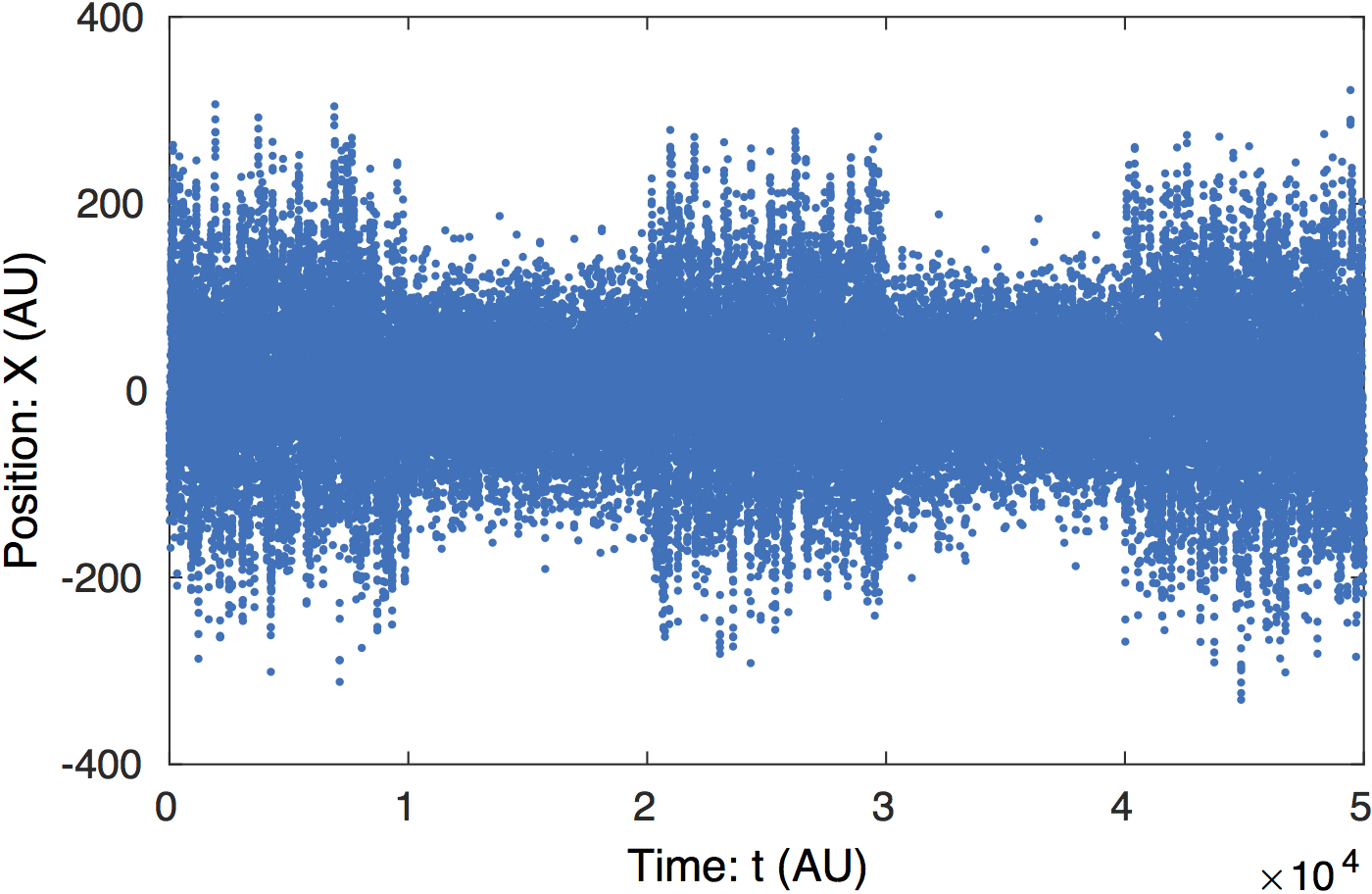Raw data.  Simulated Tethered-Particle-Motion with short and long tether lengths. The step-like transitions are clear by eye.Change-point analysis of signal. Steppi determines the positions of the change points and fits the model parameters (level means $\mu$), stiffness ($k$) and nearest neighbor coupling ($\epsilon$).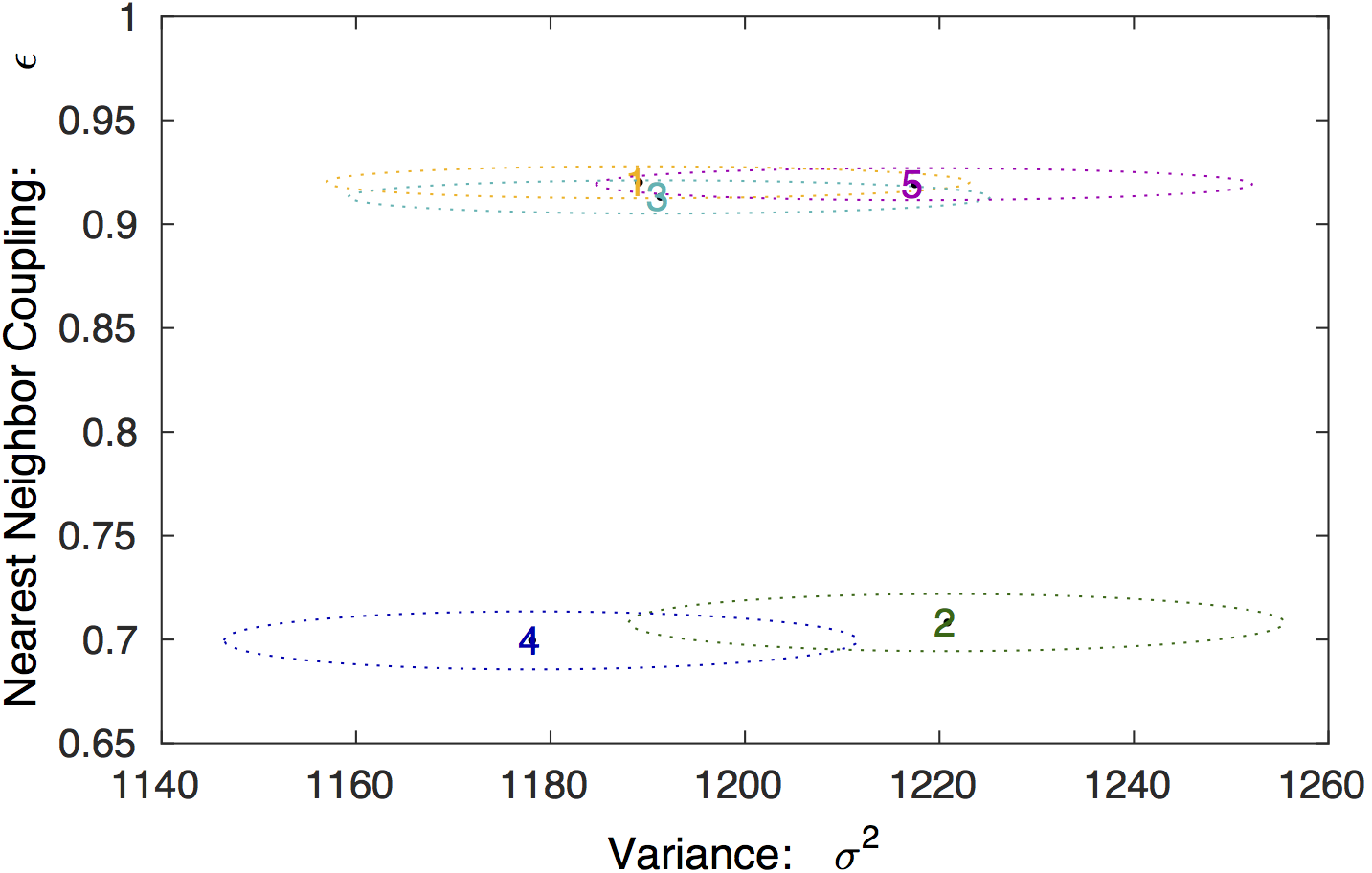Model parameter values. The 95% confidence regions for the model parameters for each state is shown, in addition to the MLE values.